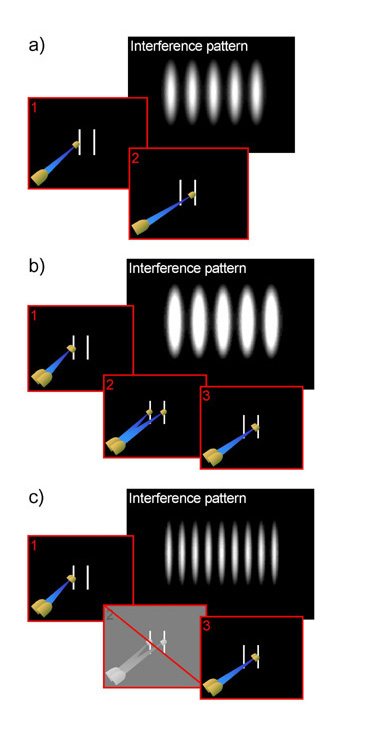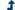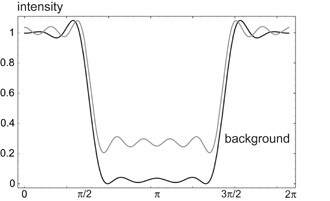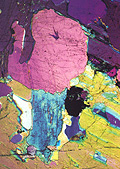### Optical Quantum Lithography

#### Contents:

Optical lithography is used in the manufacturing of microchips to etch patterns on silicon wafers. Due to diffraction, the size of these patterns is limited by the wavelength of the light used. Here, we present a new lithographic protocol that uses the quantum properties of light. With this new technique it is possible to beat the diffraction limit and, in principle, continue the miniaturization process until we reach the atomic scale.

#### Introduction

The performance of computers continues to improve at an exponential rate: according to Moore's law, every eighteen months the number of transistors on a microchip doubles. The computer industry achieves this remarkable growth in part due to the continuing miniaturizat in of the components on chips. However, there must eventually be a limit to the size of semiconductor transistors. This limit is initially determined by the production process, and ultimately by the atomic scale.The microchip industry uses optical lithography to etch patterns on silicon wafers. But the projection of light patterns has a limited precision. When the size of the features in the pattern becomes comparable to the wavelength of the light, the edges start to blur. This effect prevents the process from achieving any serious sub-wavelength resolution. Since the wavelength is much larger than the size of the molecules in the photoresist, the miniaturization process is limited by the wavelength of the light.

The blurring of the edges in optical lithography is an inherent property of the wave-nature of light. According to the nineteenth century physicist Lord Rayleigh, the size of the smallest features one can resolve with light of a particular wavelength is given by the distance between a peak and the adjacent trough in an interference pattern. Under ideal circumstances the Rayleigh limit is a quarter of the wavelength. Right now, optical lithography operates at the Rayleigh limit.

#### Incresead optical resolutionPioneering work by Yablonovich and Vrijen in 1999 , showed that it is possible to double the resolution of optical lithography by using two-photon absorption. This is accomplished by coherently superposing light fields of different frequencies and using photo-resists that are only sensitive to two-photon excitations. The use of the two-photon substrate indicates a departure from classical physics, where light is purely a wave phenomenon. The quantum description of light in terms of photons allows a whole new way of considering the origin of the Rayleigh limit, and ways to circumvent it. In January 2000, we presented a method to beat the Rayleigh limit in order to gain (in principle) arbitrary precision in lithography [2,3]. We call it quantum lithography, and here is how it works.

Suppose that, instead of using classical waves to understand the interference on a photo-sensitive substrate, we employ quantum optics. We can then describe classical interference, heuristically, in terms of single particle-like photons traveling along all possible paths. If there are only two possible paths for the photon (for example in the double-slit experiment), the interference pattern due to single-photon absorption at the surface of recombination exhibits fringes with a characteristic width that is proportional to the wavelength of the light (see the figure on the right). We can now ask the following question: what happens if we have two photons taking all possible paths?

The answer is that we obtain the same interference pattern but with twice the intensity. In the case of the double-slit experiment, the two photons either travel both through the left or right slit, or they travel through different slits. It is not surprising that we see the same interference pattern, because it should be independent of the intensity. For example, laser light contains many photons (approximately 1016 photons per second for a laser pointer), and the interference pattern does not change when the power is varied. The reason is that every photon travels independently through either the left or the right slit. The total interference pattern is therefore the sum of all single-photon interference intensity patterns.

This picture changes drastically when the photons are no longer independent. Consider for example the case of two photons: it is possible to make them travel only together, either both through the left slit, or both through the right slit, but never through different slits. These photons clearly would not be indepedent of each other; they would be path-entangled. Whichever path one photon takes gives you full information about the path of the second photon.

However, if the two photons always travel together, they behave essentially like a particle with twice the energy of the individual photons. Their two-mode quantum state is given by |2,0\rangle_{ab}+|0,2\rangle_{ab}, where a and b are the different paths. Such a pseudo-particle must have its own de Broglie wavefunction. A single photon has momentum $p = h/\lambda$, where $h$ is Planck's constant and $\lambda$ the wavelength of the light. The momentum of the pseudo-particle is then $2p$, and the de Broglie wavelength is $\lambda' = h/(2p)$. This wavelength is half as long as the wavelength of the light: $\lambda = 2\lambda'$. The fringes of the interference pattern are therefore also half as narrow. To see this effect, the surface needs a suitable resist that is sensitive to two-photon absorption.

#### N-photon lithographyConceptually, it is now a small step to find an interference pattern that is $N$ times as narrow as a single-photon pattern. Suppose we create a pseudo-particle consisting of $N$ photons, that is, a quantum state $|N,0\rangle_{ab}+|0,N\rangle_{ab}$. The total momentum is then given by $Np$, and the effective de Broglie wavelength is $\lambda/N$. This leads to an interference pattern where the minimal distance from a peak to the adjacent trough is proportional to $\lambda/N$. This shows that if you use quantum mechanics to create correlated many-photon states you can beat the classical limit set by Rayleigh.

The distance on the substrate can be represented by a length parameter $x$ that is directly related to a phase: $\phi = 2\pi x/\lambda$. Thus, the minimal unambiguous phase shift we can measure corresponds to a shift $\Delta x$ from a bright spot to a dark spot on the interference pattern, a criterion analogous to Rayleigh's. Thus, both physically and mathematically, quantum lithography is closely related to quantum-limited measurements of phase.

When photons behave like independent particles, for example in laser light, the minimal phase precision is given by the so-called shot-noise limit $1/\sqrt{\bar{n}}\propto 1/\sqrt{I}$. Here, $\bar{n}$ is the average number of photons in a coherent state, proportional to the classical intensity $I$. This precision is very much a classical limit, just like the Rayleigh limit turned out to be. When we use the path-entanglement of $N$-photon pseudo-particles, as in quantum lithography, we can boost the precision in phase measurements to the fundamental Heisenberg limit of $1/N$, instead of $1/\bar{n}$. These two values can be compared when we consider fields with equal energy $E = \bar{n}\, hc/\lambda = N\, hc/\lambda$, where $c$ is the speed of light in vacuum. The use of optimal quantum states thus yields a quadratic improvement in the precision of phase measurements. However, in lithography, exotic quantum states allow us to surpass the classical limits entirely.

#### Arbitrary patternsSo far, we only considered the etching of closely spaced lines with multi-photon pseudo-particles. However, lithographic applications need more interesting patterns. For simplicity, consider only one dimension. Any intensity pattern, as a function of the displacement on the substrate, can be decomposed into its Fourier components. These components are trigonometric functions of $x$: $\cos Nx$ and $\sin Nx$. Except for an easily implemented phase shift, the intensity patterns obtained with quantum lithography are exactly these Fourier components. As a consequence, by successive exposure of a suitable multi-photon resist with $\cos Nx$ and $\sin Nx$ intensity patterns, quantum lithography can in principle approximate any pattern in one dimension.There is a catch, however. Since this procedure involves subsequent illumination of the substrate with patterns of different line spacing, once a region is exposed this cannot be undone. The result is an intensity pattern that matches the required pattern up to a constant background exposure. Depending on the application, this might or might not be an impediment.

Alternatively, we can use more exotic quantum states than the so-called noon states ($|N,0\rangle+|0,N\rangle$) to create more interesting patterns. By turning interference to our advantage, it is possible to remove the constant background exposure from the final pattern. One way to achieve this, is to generalize the concept of the pseudo-particle. Instead of $N$ photons traveling along path $a$ (or path $b$) and nothing along path $b$ (or path $a$), we can split off $m$ photons from one path, and send them along the other. The correponding state is then $|N-m,m\rangle_{ab} + |m,N-m\rangle_{ab}$. This will increase the spacing in the interference pattern. If the total number of photons $N$ is fixed, we can make a whole family of states that are labelled by $m$, the number of photons that are split off. Since $N$ is fixed, all these states interfere with each other on the substrate when they are used in a superposition. Hence such exotic superposition states may reduce the exposure in unwanted regions to zero.

By introducing this extra degree of freedom we have gained the relative quantum amplitudes of the different states $|N-m,m\rangle + |m,N-m\rangle$ to play with. We have shown numerically that with this interference method it is possible to approximate a square well intensity pattern without the uniform background exposure (see figure \ref{fig2}). The drawback is that we need quite complicated quantum states, and there is no straightforward formula to relate the required patterns to the relative amplitudes of the states.

A third method to create non-trivial, sub-wavelength patterns with quantum lithography was proposed by Bj\"ork and co-workers in 2001. They used the extra degrees of freedom of the relative amplitudes in these multi-photon states to tailor a sub-wavelength pixel. The advantage over our method is that Bj\"ork et al.  do not need an algorithm relating the desired pattern to a photonic quantum state. However, pixellation of large areas might take a very long time . In addition, the state that is required to write a single pixel is highly non-classical, and at least as difficult to make as the multi-photon superposition states described above.

#### Outlook and concusionsThese are not the only problems that implementations of quantum lithography have to overcome. Until now we have implicitly assumed that we have photo-resists that are sensitive to $N$ photons. However, if more than two photons are used, such materials are not yet developed.

Another difficulty is the creation of the necessary quantum states. It is well known that the state $|2,0\rangle+|0,2\rangle$ can be made with a beam splitter . When two identical photons enter a beam splitter, one from each side, the two photons will either both emerge from one side of the beam splitter or the other. Due to destructive interference they will never emerge from different sides, which means that we effectively created a two-photon pseudo-particle. For noon states, the creation process is not that simple. When we are restricted to linear optics, Lee et al. have shown that one must employ projective measurements via post-selection to produce more complicated states [8,9]. Such a restriction would lead to an exponential deterioration in the probability of preparing the desired states.

Hypothetically, let's suppose that we eventually overcome all these difficulties and the lithographic procedure is no longer the limiting factor in the miniaturization of microchips. On that happy day the feature size will only be limited by the atomistic nature of matter. However, at those scales electrons no longer behave like classical currents, and various quantum effects will add noise to classical computers that are this small. As we approach the fundamental limits in our ability to manipulate matter and to process information, these quantum effects may in turn be harnessed into quantum computers.

#### References1. E. Yablonovich and R.B. Vrijen, Opt. Eng. 38, 334-338 (1999).
2. A.N. Boto, P. Kok, D.S. Abrams, S.L. Braunstein, C.P. Williams, and J.P. Dowling, Phys. Rev. Lett 85, 2733 (2000).
3. P. Kok, A.N. Boto, D.S. Abrams, C.P. Williams, S.L. Braunstein, and J.P. Dowling, Phys. Rev. A 63, 063407 (2001).
4. G. Bjork, L.L. Sanchez-Soto, and J. Soderholm, Phys. Rev. Lett. 86, 4516 (2001).
5. This is the main reason why manufacturers do not use near-field lithography; the evanescent waves of the electromagnetic field are not bounded by the Rayleigh limit, but the time it takes to scan an area the size of a microchip is unpractical .
6. V. Sandoghdar, Physics World September 2001 pp. 29-33.
7. C.K. Hong, Z.Y. Ou, and L. Mandel, Phys. Rev. Lett. 59, 2044 (1987).
8. H. Lee, P. Kok, N.J. Cerf, and J.P. Dowling, Phys. Rev. A 65, 030101R (2002).
9. P. Kok, H. Lee, and J.P. Dowling, Phys. Rev. A 65, 052104 (2002).